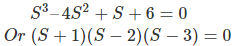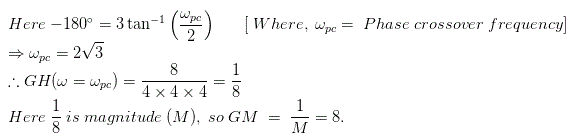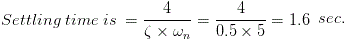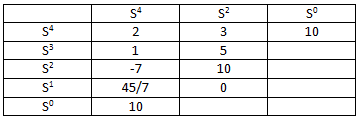# MCQs on Control Systems

##### Page 9 of 23. Go to page 1 2 3 4 5 6 7 8 9 10 11 12 13 14 15 16 17 18 19 20 21 22 23
01․ In a control system, an integrator is represented by
s.
s2.
1 / s2.
1 / s.

Intergrator is always represented by ( 1 /s ) i.e 1 pole at the origin. Differentiator is represented by s i.e 1 zero at the origin.

02․ For a lead compensator, the poles lie:
on LHS before zero.
on origin.
on LHS.
on RHS.

In lead compensator network pole lies on LHS and pole lies before zero. Zero lies before pole on LHS in the case of the lag compensator.

03․ The phase of lead compensator of the system G ( s ) = ( s + a ) / ( s + b ) is at its maximum at:
ab.
√( ab ).
√( a / b).
a / b.

The phase of this lead compensator system is maximum at √(ab).

04․ Number of roots in left hand half of s plane if characteristic equation is s3 - 4s2 + s + 6 = 0 ?
1.
2.
3.
0.So there is only one pole on the left half of s plane.

05․ The value of gain margin of the system having G ( s )H ( s ) = 8 / ( s + 2 )3 is
8.
2.
6.
4.06․ The second order system defined by 25/(s2 + 5s + 25) is given a step input. The time taken for the output to settle with in 2 % of input is
1.6 sec.
1.2 sec.
2 sec.
0.4 sec.07․ The amplitude spectrum of a Gaussian pulse is
Uniform.
A sine function.
Gaussian.
Impulse function.

The amplitude spectrum of a Gaussian pulse is always Gaussian.

08․ The characteristic equation of a feedback control is 2s4 + s3 + 3s2 + 5s + 10 = 0. The no of roots in the right half of the s-plane is
2.
3.
4.
0.

Applying Routh-Hurwitz criterion as shown below,We observe that the sign of the first column changes twice, so the number of roots on the right hand side of S plane = 2.

09․ If a body has identical properties all over, it is known as
elastic.
homogeneous.
isotropic.
none of them.

Homogeneity is the property of having all parts the same or similar in type.

10․ A function of one or more variables which conveys information about the nature of physical phenomenon is called
signal.
interference.
system.
noise.

Signal is also defined as any physical quantity that varies with time, space or any other independent variable.

<<<7891011>>>